Ex 11.4

Chapter 11 Class 7 Perimeter and Area
Serial order wise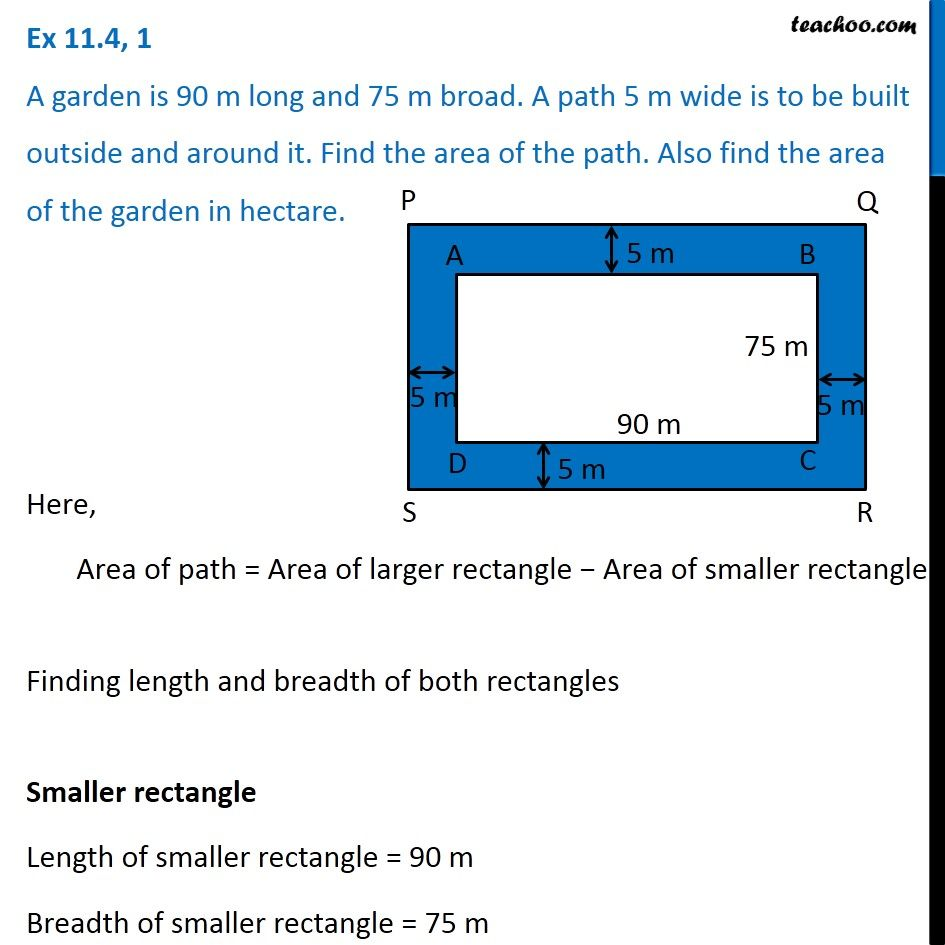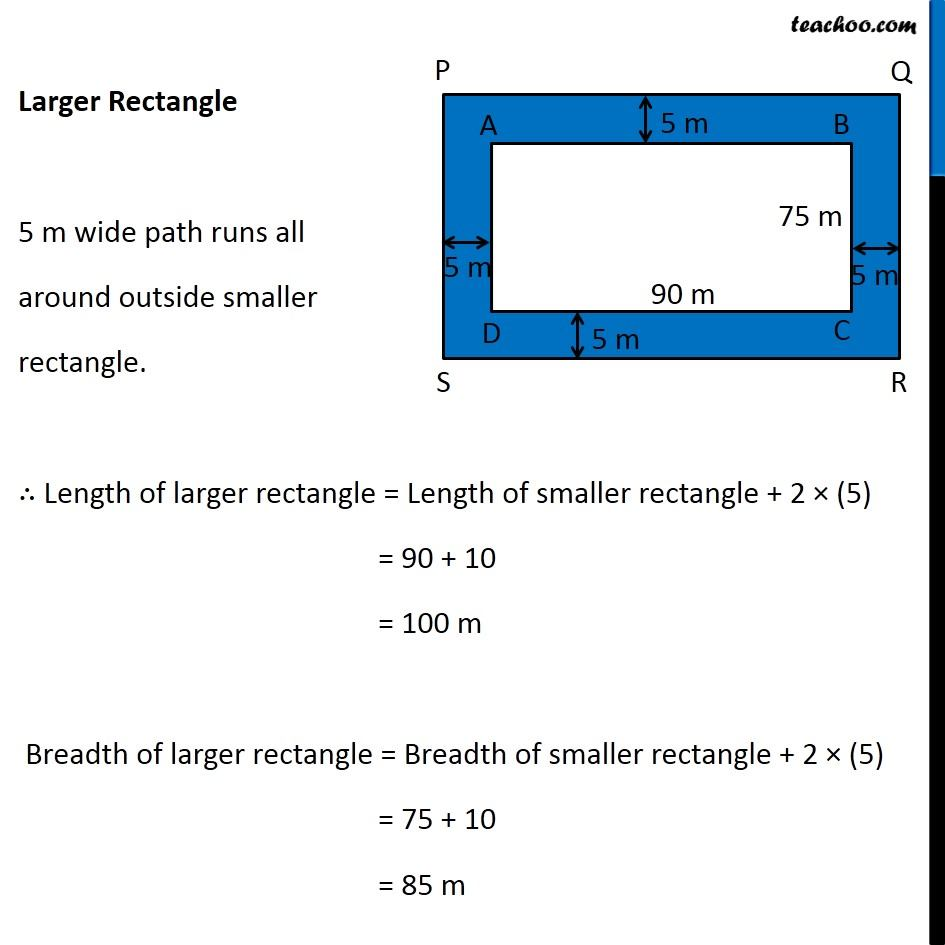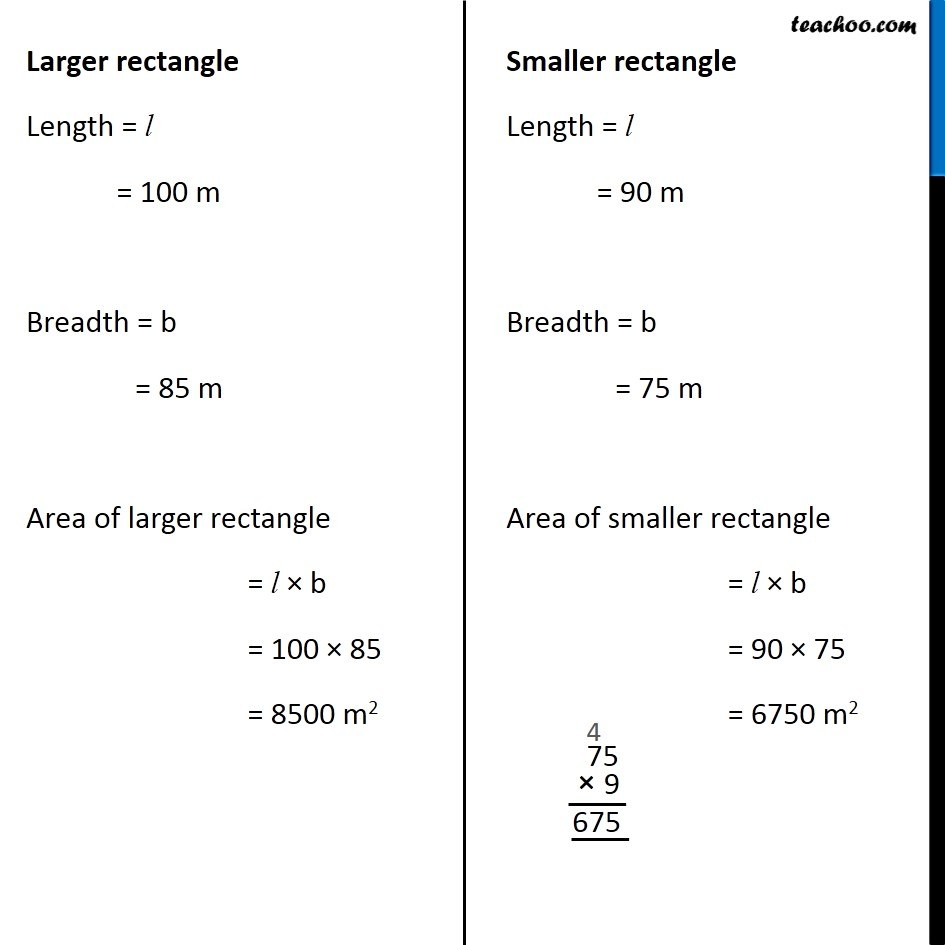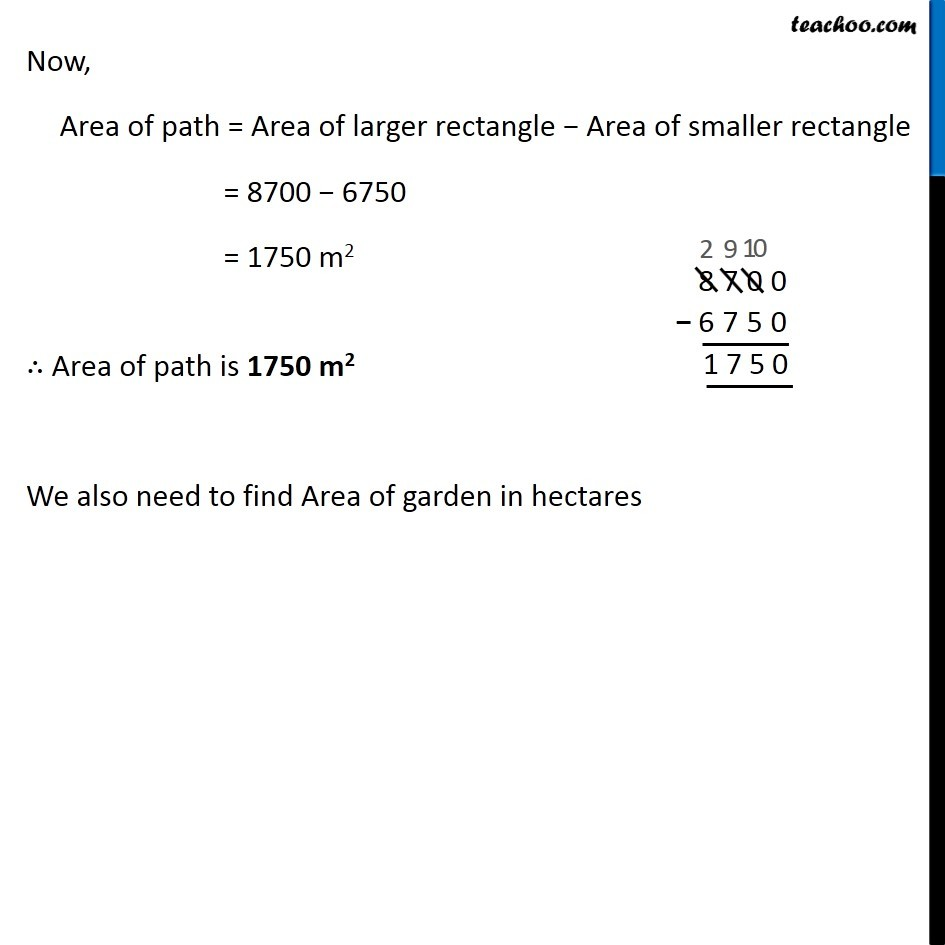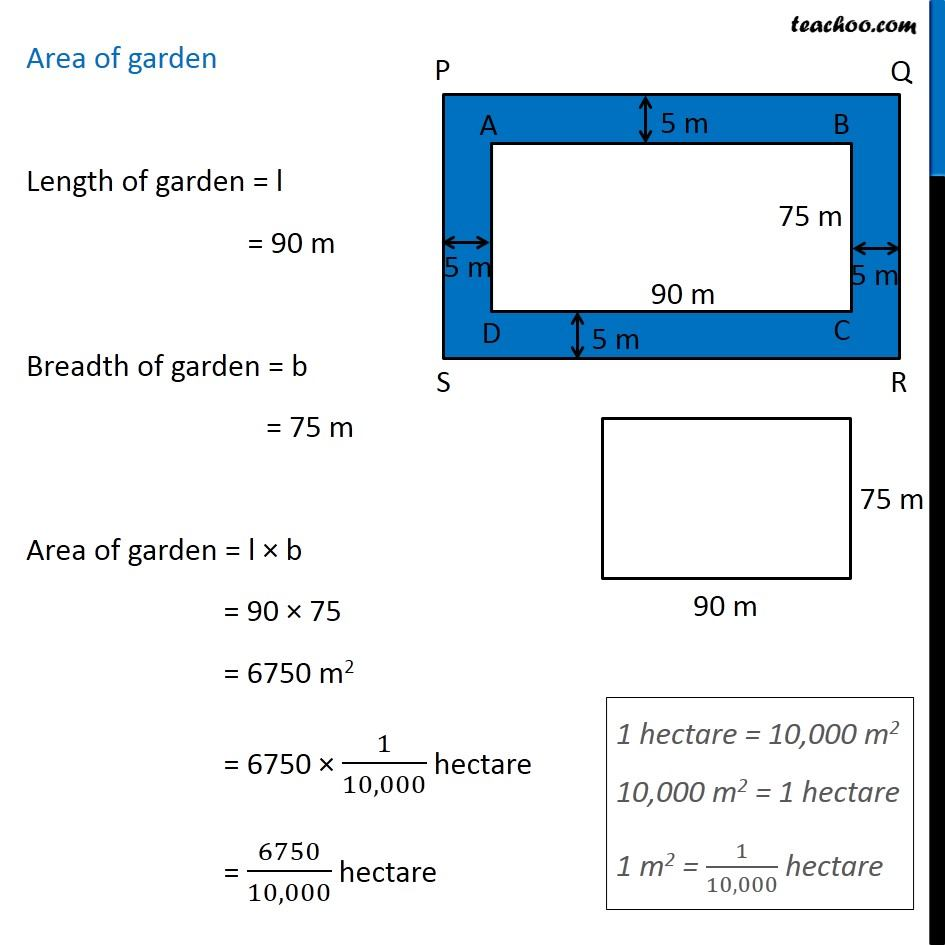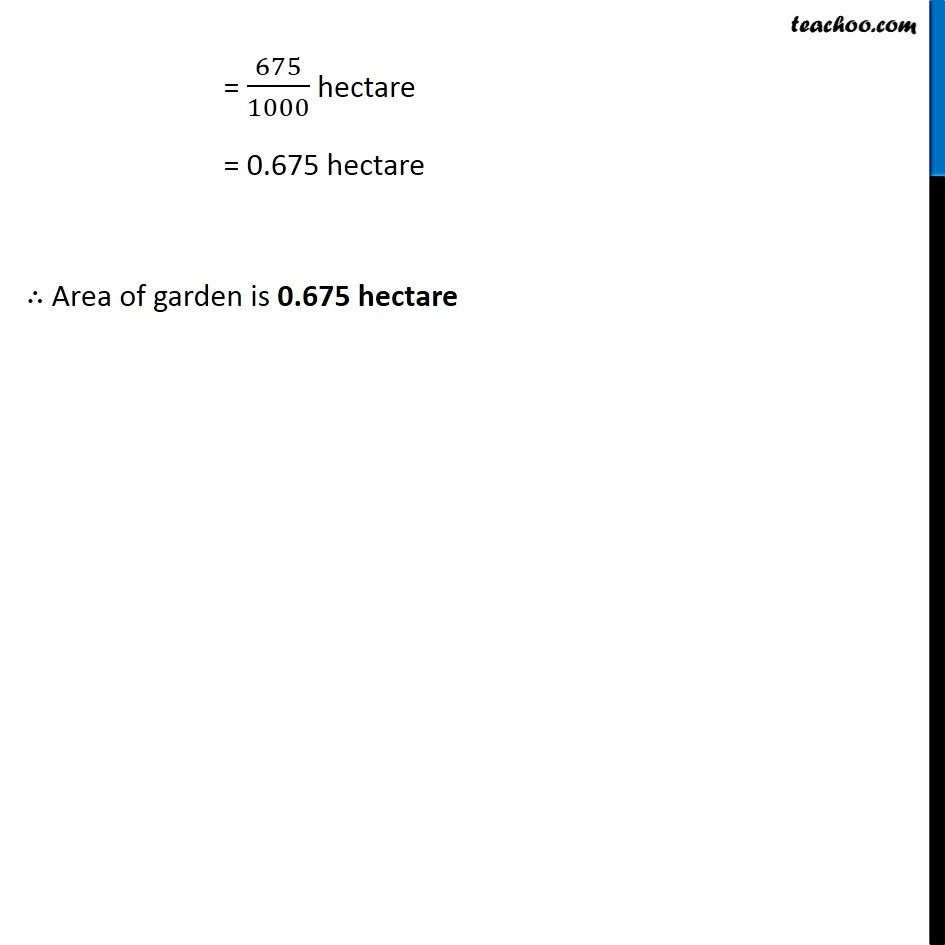Get live Maths 1-on-1 Classs - Class 6 to 12

### Transcript

Ex 11.4, 1 A garden is 90 m long and 75 m broad. A path 5 m wide is to be built outside and around it. Find the area of the path. Also find the area of the garden in hectare. Here, Area of path = Area of larger rectangle − Area of smaller rectangle Finding length and breadth of both rectangles Smaller rectangle Length of smaller rectangle = 90 m Breadth of smaller rectangle = 75 m Larger Rectangle 5 m wide path runs all around outside smaller rectangle. ∴ Length of larger rectangle = Length of smaller rectangle + 2 × (5) = 90 + 10 = 100 m Breadth of larger rectangle = Breadth of smaller rectangle + 2 × (5) = 75 + 10 = 85 m Larger rectangle Length = l = 100 m Breadth = b = 85 m Area of larger rectangle = l × b = 100 × 85 = 8500 m2 Smaller rectangle Length = l = 90 m Breadth = b = 75 m Area of smaller rectangle = l × b = 90 × 75 = 6750 m2 Now, Area of path = Area of larger rectangle − Area of smaller rectangle = 8700 − 6750 = 1750 m2 ∴ Area of path is 1750 m2 We also need to find Area of garden in hectares Area of garden Length of garden = l = 90 m Breadth of garden = b = 75 m Area of garden = l × b = 90 × 75 = 6750 m2 = 6750 × 1/10,000 hectare = 6750/10,000 hectare 1 hectare = 10,000 m2 10,000 m2 = 1 hectare 1 m2 = 1/10,000 hectare = 675/1000 hectare = 0.675 hectare ∴ Area of garden is 0.675 hectare